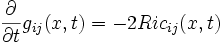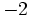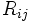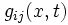# Ricci flow

## Definition

The Ricci flow is a flow on the space of all Riemannian metrics on a given differential manifold. Note that the space of all Riemannian metrics is a Banach manifold. More precisely, the space of Riemannian metrics is contained inside the space of all sections of the second tensor power of the cotangent bundle, because a Riemannian metric is after all a$(0,2)$-tensor.

The Ricci flow is governed by the following differential equation:$\frac{\partial}{\partial t} g_{ij}(x,t) = -2Ric_{ij} (x,t)$

In other words, the rate at which the Riemannian metric tensor is changing with time, is$-2$ times the Ricci curvature tensor at that time (both are$(0,2)$-tensors).

## Facts

The motivation behind the Ricci flow is to evolve any given Riemannian metric on the differential manifold to a constant-curvature metric, or at least, to an Einstein metric. In dimension 2, the constant-curvature metrics are the same as the Einstein metrics.

### Stationary points

A point is stationary for this flow if and only if it is: Ricci-flat metric

For a Ricci-flat metric, the equation becomes:$\frac{\partial}{\partial t} g_{ij}(x,t) = 0$

Hence, the Ricci-flat metrics remain invariant under the Ricci flow (in fact, these are precisely the stationary points under the Ricci flow).

### Effect on Einstein metrics

Notice that in an Einstein metric,$R_{ij}$ is proportional to$g_{ij}$. So if we consider the above Ricci flow for an Einstein metric, we get:$\frac{\partial}{\partial t} g_{ij}(x,t) = -2\lambda g_{ij} (x,t)$

Here$\lambda$ is the cosmological constant of the Einstein metric.

This simply describes an exponential decay of$g_{ij}(x,t)$ with time. Hence, the conformal class of the Riemannian structure does not change. Hence, Einstein metrics, while not invariant under the Ricci flow, are invariant upto the conformal structure.

On the other hand, the volume-normalized Ricci flow actually does have Einstein metrics as the stationary points.